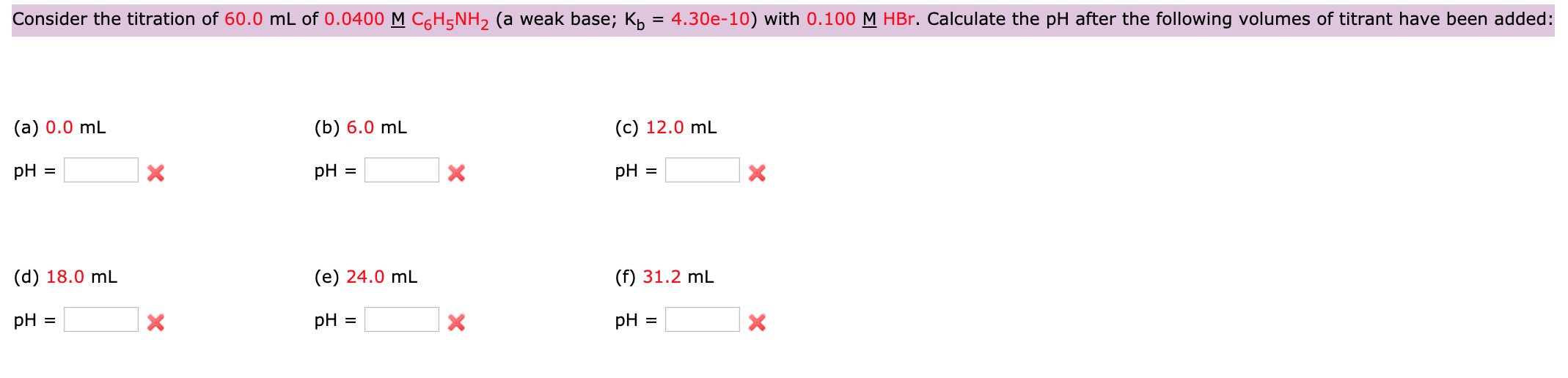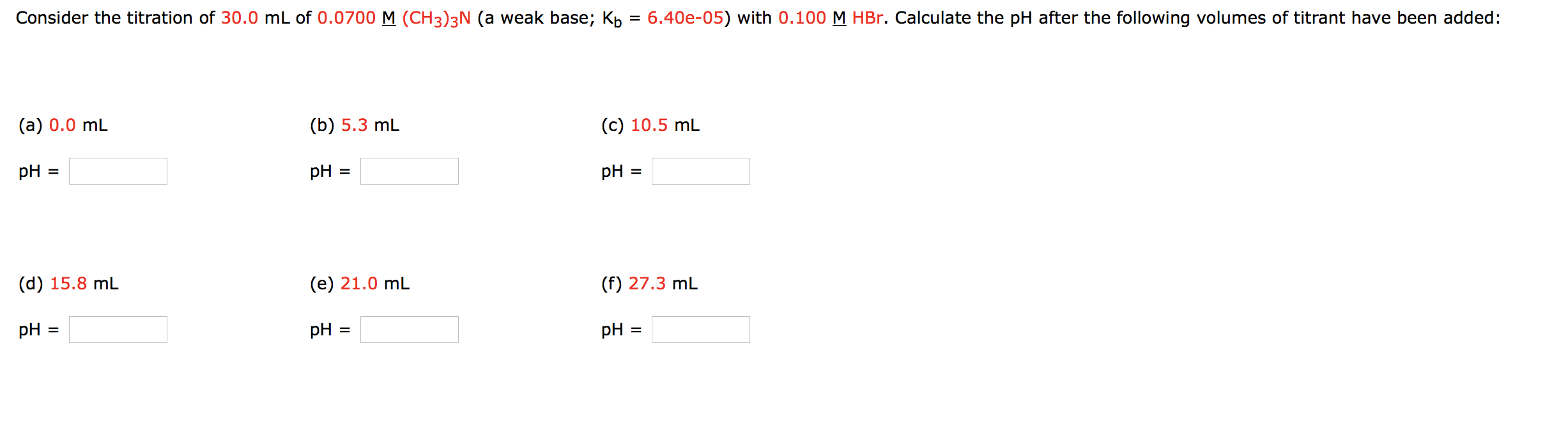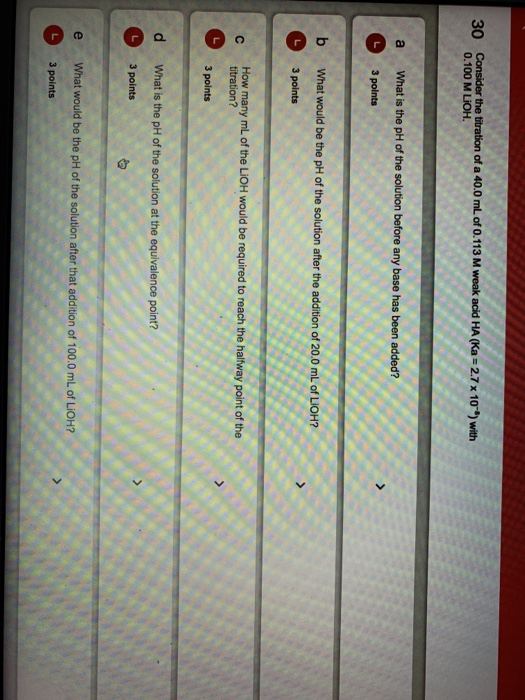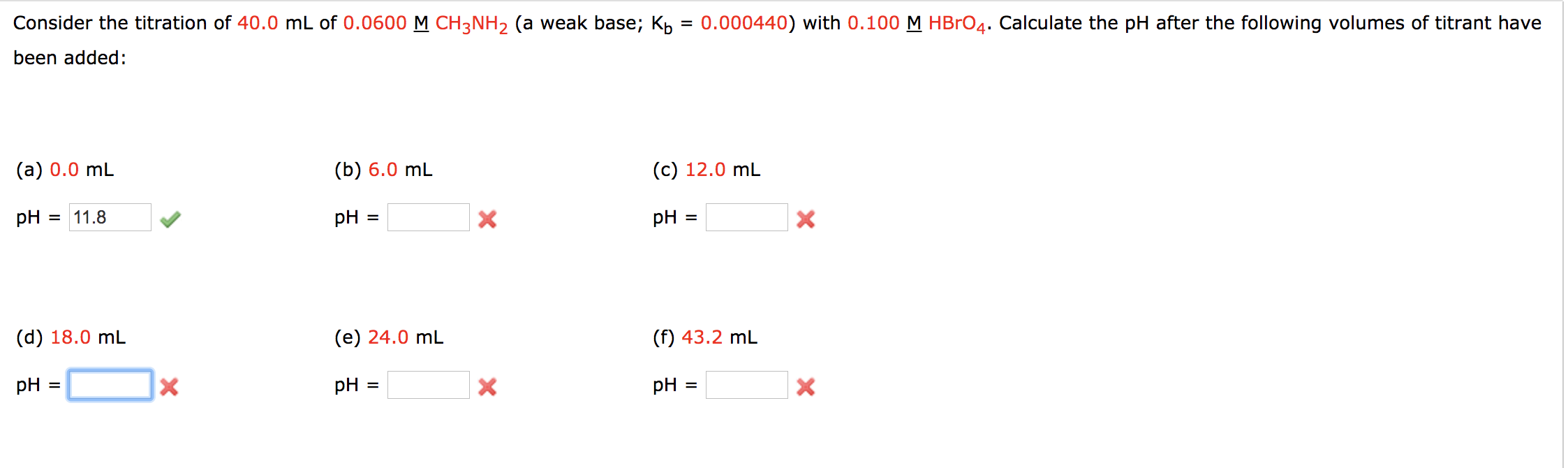# Consider the titration of 30.0 mL of 0.283 M weak base B (Kb = 1.3 x...

Consider the titration of 30.0 mL of 0.283 M weak base B (Kb = 1.3 x 10⁻¹⁰) with 0.150 M HI. What would be the pH of the solution after the addition of 100.0 mL of HI?

Given:
M(HI) = 0.15 M
V(HI) = 100 mL
M(B) = 0.283 M
V(B) = 30 mL

mol(HI) = M(HI) * V(HI)
mol(HI) = 0.15 M * 100 mL = 15 mmol

mol(B) = M(B) * V(B)
mol(B) = 0.283 M * 30 mL = 8.49 mmol

We have:
mol(HI) = 15 mmol
mol(B) = 8.49 mmol

8.49 mmol of both will react
excess HI remaining = 6.51 mmol
Volume of Solution = 100 + 30 = 130 mL
[H+] = 6.51 mmol/130 mL = 0.0501 M

use:
pH = -log [H+]
= -log (5.008*10^-2)
= 1.3004

#### Earn Coin

Coins can be redeemed for fabulous gifts.

Similar Homework Help Questions
• ### Consider the titration of 60.0 mL of 0.0400 M C6H5NH2 (a weak base; Kb = 4.30e-10)...Consider the titration of 60.0 mL of 0.0400 M C6H5NH2 (a weak base; Kb = 4.30e-10) with 0.100 M HBr. Calculate the pH after the following volumes of titrant have been added: Consider the titration of 60.0 mL of 0.0400 M C H5NH2 (a weak base; Kb = 4.30e-10) with 0.100 M HBr. Calculate the pH after the following volumes of titrant have been added: (a) 0.0 mL (C) 12.0 mL (b) 6.0 mL pH = pH = x pH...

• ### Consider the titration of 30.0 mL of 0.0700 M (CH3)3N (a weak base; Kb = 6.400-05) with 0.100 M HBr. Calculate the pH a...Consider the titration of 30.0 mL of 0.0700 M (CH3)3N (a weak base; Kb = 6.400-05) with 0.100 M HBr. Calculate the pH after the following volumes of titrant have been added: (a) 0.0 mL (b) 5.3 mL (c) 10.5 mL pH = pH = pH = (d) 15.8 ml (e) 21.0 mL (f) 27.3 mL pH = pH = pH =

• ### Consider the titration of 70.0 mL of 0.0300 M C2H5NH2 (a weak base; Kb = 0.000640)...

Consider the titration of 70.0 mL of 0.0300 M C2H5NH2 (a weak base; Kb = 0.000640) with 0.100 M HI. Calculate the pH after the following volumes of titrant have been added: (a) 0.0 mL pH =   (b) 5.3 mL pH =   (c) 10.5 mL pH =   (d) 15.8 mL pH =   (e) 21.0 mL pH =   (f) 23.1 mL pH =

• ### Consider the titration of 80.0 mL of 0.0200 M NH3 (a weak base; Kb = 1.80e-05)...

Consider the titration of 80.0 mL of 0.0200 M NH3 (a weak base; Kb = 1.80e-05) with 0.100 M HI. Calculate the pH after the following volumes of titrant have been added: (a) 0.0 mL pH =   (b) 4.0 mL pH =   (c) 8.0 mL pH =   (d) 12.0 mL pH =   (e) 16.0 mL pH =   (f) 20.8 mL pH =

• ### 30 Consider the titration of a 40.0 mL of 0.113 M weak acid HA (Ka=2.7 x...30 Consider the titration of a 40.0 mL of 0.113 M weak acid HA (Ka=2.7 x 10) with 0.100 M LIOH. a What is the pH of the solution before any base has been added? 3 points b What would be the pH of the solution after the addition of 20.0 mL of LIOH? 3 points How many mL of the LiOH would be required to reach the halfway point of the titration? 3 points d What is the pH...

• ### Consider the titration of 40.0 mL of 0.0600 M CH3NH2 (a weak base; Kb = 0.000440)...Consider the titration of 40.0 mL of 0.0600 M CH3NH2 (a weak base; Kb = 0.000440) with 0.100 M HBrO4. Calculate the pH after the following volumes of titrant have been added: (a) 0.0 mL (b) 6.0 mL pH = (C) 12.0 mL pH = pH = 11.8 * x (d) 18.0 ml (e) 24.0 ml (f) 43.2 ml pH = pH = pH =

• ### 38. Consider the titration of 80.0 mL of 0.0200 M C6H5NH2 (a weak base; Kb =...

38. Consider the titration of 80.0 mL of 0.0200 M C6H5NH2 (a weak base; Kb = 4.30e-10) with 0.100 M HNO3. Calculate the pH after the following volumes of titrant have been added: (a) 0.0 mL pH = ________ (b) 4.0 mL pH = ___________ (c) 8.0 mL pH = ________ (d) 12.0 mL pH = __________ (e) 16.0 mL pH = __________ (f) 24.0 mL pH = _________

• ### Consider the titration of 20.0 mL of 0.0800 M C6H5NH2 (a weak base; Kb = 4.30e-10)...

Consider the titration of 20.0 mL of 0.0800 M C6H5NH2 (a weak base; Kb = 4.30e-10) with 0.100 M HIO4. Calculate the pH after the following volumes of titrant have been added: (a) 0.0 mL pH = (b) 4.0 mL pH = (c) 8.0 mL pH = (d) 12.0 mL pH = (e) 16.0 mL pH = (f) 22.4 mL pH=

• ### Consider the titration of 80.0 mL of 0.0200 M C6H5NH2 (a weak base; Kb = 4.30e-10)...

Consider the titration of 80.0 mL of 0.0200 M C6H5NH2 (a weak base; Kb = 4.30e-10) with 0.100 M HBrO4. Calculate the pH after the following volumes of titrant have been added: (a) 0.0 mL pH =   (b) 4.0 mL pH =   (c) 8.0 mL pH =   (d) 12.0 mL pH =   (e) 16.0 mL pH =   (f) 25.6 mL pH =

• ### A. Match each type of titration to its pH at the equivalence point. Weak acid, strong base Strong acid, strong base...

A. Match each type of titration to its pH at the equivalence point. Weak acid, strong base Strong acid, strong base Weak base, strong acid pH less than 7 pH equal to 7 pH greater than 7 B. A 56.0 mL volume of 0.25 M HBr is titrated with 0.50 M KOH. Calculate the pH after addition of 28.0 mL of KOH. C. Consider the titration of 50.0 mL of 0.20 M NH3 (Kb=1.8 x 10^-5) with 0.20 M HNO3....

Free Homework App